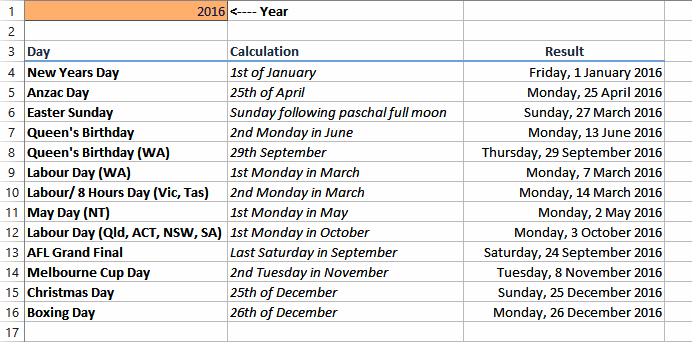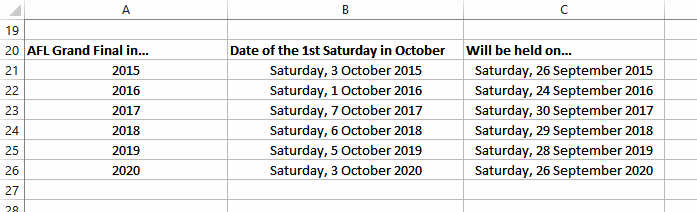Close

## How About A Date Part 2

Note: This is the second of a two part series. You can view part 1 here.

In our last blog we were looking for dates - no, not the romantic ones - that occur annually. We set about trying to figure out to calculate them for future years. We looked at simple formulas for creating fixed dates such as New Years Day and Christmas, and then got into the murkier dates that fall on the first Monday or the second Tuesday in a month. In this blog we plunge the murky depths even further and look at calculating dates that fall on the last particular day of a month as well as the one date that defies us just creating a simple formula – Easter Sunday!

### The AFL Grand Final

Let's re-examine our sample of dates that we want to calculate. They range from quite simple to extremely complex. But there is only one that falls on the last day of a month - the Australian Rules Football League Grand Final. That most venerable of sporting days in the Victorian calendar is held on the last Saturday in September.The formula we've come up for this is rather sneaky (actually, it's not ours but one based upon - but not exactly the same as - that shown in an old copy of John Walkenbach's Microsoft Excel 2000 Formulas book which we've got lying around).

John comes up with a brilliant idea for this type of formula that uses a bit of lateral thinking. In the previous blog we derived a formula that calculates the nth number of a day in a month (e.g. the first Tuesday, the third Monday, etc). John's idea is that to find the last particular day of the month, in our case a Saturday, we simply find the first Saturday of the next month then subtract 7 days - brilliant!

So let's begin by finding the first Saturday in October (not September). Actually this is a bit of a recap from the last blog.

Remember that in Excel the days of the week are given an index number starting from Sunday (1) through to the following Saturday (7). In this scheme Saturday is day number 7 in the week. You can find the index number for any given date using the WEEKDAY() function which requires the serial number of the date.

So the logic for our AFL Grand Final calculation is to find the date for the first of October then add the number of days to the first Saturday to that date. The first part is achieved by using the DATE() function again.

Finding the number of days to the first Saturday requires a bit more work. We do this with the following logic:

1. If the weekday index number of October 1 is less than the index number for Saturday (7), we take the index number of October 1 away from 7 and add 7 (the index number for Saturday)
2. If the weekday index number of October 1 is greater than the index number for Saturday, we take the index number for October 1 away from 7 (the index number for Saturday)

To express this in pseudo-Excel function language we'd use an IF() function as follows:

```IF(7 < WEEKDAY(October 1, 2016), 7 - WEEKDAY(October 1, 2016) + 7, 7 - WEEKDAY(October 1, 2016))
```

October 1, 2016, actually falls on a Saturday which gives us an index number of 7. Placing the index number into this formula for 2016 gives us:

```IF(7 < 7, 7 - 7 + 2, 7 - 7)
```

which will return 0 (because 7 can't be less than 7, therefore we revert to the second part of the if statement where 7 -7 equals 0).

So we know that the first Saturday in October 1, 2016, actually is the first day of the month. To determine the last Saturday of September we simply deduct 7 days.

In the above screen we've used the following formula (assuming the year is in cell A1):

```=DATE(A1,10,1)+IF(7 < WEEKDAY(DATE(A1, 10, 1)), 7 - WEEKDAY(DATE(A1, 10, 1)) + 7, 7 - WEEKDAY(DATE(A1, 10, 1))) - 7
```

Aha, if we extrapolate that over a few years we get the following:So now we know when to start putting the beer on ice!

Oops, there's a bit of a gotcha with this example. In 2015 the AFL Grand final is actually being held on October 3! Is our formula wrong? No, it's that the whole season has slipped one week so as to not clash with the World Cup of Cricket. Oh well, it pays to check the results of your spreadsheets!

### Easter Sunday

#### Understanding Computus

Computus is the name given to the calculation that is used to determine the calendar date of Easter. The problem with Easter is that it is based on a calendar rather than an astronomical event. Since Christians have worked to two calendars over the centuries (the Julian and Gregorian calendars) calculating Easter has become a nightmare, and varies depending upon the western and eastern Christian religions.

Cutting to the chase, in general Easter falls on the Sunday following the full moon that follows the northern spring equinox, known as the paschal (pronounced "PAS-KUL") full moon. Easter Sunday is the date that Christians celebrate Christ's resurrection. According to the Astronomical Association of South Australia (ASSA):

"The aim of the Easter Dating Method is to maintain, for each Easter Sunday, the same season of the year and the same relationship to the preceding astronomical full moon that occurred at the time of [Christ's] resurrection in 30 A.D."

The ASSA have done a lot of work in this area and on their web site you'll find the dates for Easter Sunday past, present and well into the future. They also have tables that you can use to calculate the dates yourself using a calculator and algorithms you can use to calculate the date using a computer program. (see for more information).

But this whole process of calculating Easter Sunday has annoyed spreadsheet nerds for decades. Yes, you can create complex calculations and algorithms that require multiple tables in a spreadsheet – but where's the fun in that?

So in 1999 a German spreadsheet user by the name of Hans Herber decided to hold a competition online asking people to develop the shortest possible formula they could that would calculate the date for Easter Sunday between the years 1900 and 2078. If you're good at German you'll find these formulas and other Excel goodies at .

The winning formula came from Norbert Hetterich of Germany with this:

```=FLOOR(DAY(MINUTE(J / 38) / 2 + 56) &"/5/" &J, 7) - 34
```

(the letter J represents a named cell containing the year - a sneaky way of reducing the size of your formula!)

You can get a good run-down on this and the other following formulas at .

Some of the other formulas are shown below:

```=DOLLAR(("4/"&J) / 7 + MOD(19 * MOD(J, 19) - 7, 30) * 14%,) * 7 - 6
```

which came 2nd by Thomas Jansen.

```=FLOOR(DATE(J, 3, MOD(18.37 * MOD(J, 19) - 6, 29)), 7) + 29
```

which came 3rd by Roger Friedrich.

We're not even going to try and explain how these formulas work. Even noted Excel MVP's online such as Jerry Latham, Chip Pearson, and George Simms struggle to do this.

The one voted most interesting was presented by Prasad DV. Try and work this one out!

```=TRUNC(DATE(J, 7, -CODE(MID("NYDQ\JT_LWBOZER]KU`", MOD(J, 19) + 1, 1))) / 7) * 7 + 8
```

#### Further References

There's a lot of stuff out there on calculating Easter Sunday and a quick Google search will reveal a lot of them. Wikipedia has an excellent (although brain numbing) overview. For a run down on the Excel calculations above you can't go past the Contextures web site () while a thorough examination is done on the Astronomical Association of South Australia's web site ().

Good luck!